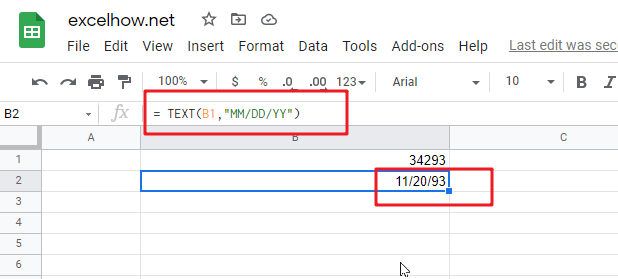This post will guide you how to use Google Sheets TEXT function with syntax and examples.

## Description

The Google Sheets TEXT function converts a numeric value into text string with a specified format.

The TEXT function can be used to convert a number formatted as text according to a specified format in google sheets.  The purpose of this function is to convert a number to text in a number format. and its returned value is a number as text in the given format.

The TEXT function is a build-in function in Google Sheets and it is categorized as a Text Function.

## Syntax

The syntax of the TEXT function is as below:

= TEXT (value, Format code)

Where the TEXT function arguments are:

• number -This is a required argument. The value you want to format.
• Format code– This is a required argument. The format code that you want to apply.

The “`Format Code`” can be used in the excel Text function are shown in the below table.

 Format Code Description Examples 0 only display digits in its place #.00 – Forces the function to display two decimal places =Text(34.234,”\$##.00″) result: \$34.23 # Display the placeholder =Text(4.527,”#.##) result: 4.53 . the position of Decimal Point =Text(342.2,”0.00″) result: 342.20 d Day of the month or day of week d- one or two digit number (1-31) dd- two digit number (01-31) ddd-abbreviated day of week (Mon to Sun) dddd-full name of day of week(Monday to Sunnday) =Text(TODAY(),”DDDD”) result: Monday m The Month of the Year m- one or two digit number mm-two digit number mmm-abbreviated month(Jan to Dec) mmmm-full name of month(January to December)) =Text(TODAY(),”MM/DD/YY”) result:11/06/17 y year yy-two digit representation of year(e.g.01,17) yyyy-four digit representation of year(e.g. 2001,2017) =Text(TODAY(),”MM/DD/YY”) result:11/06/17 h Hour h-one or two digit number (e.g. 1,23) hh-two digit number (e.g. 01,23) =Text(14:16,”hh:mm”) result: 14:16 m Minute m-one or two digit representation (e.g. 1,59) mm-two digit representation (e.g. 01,59) =Text(14:16,”hh:mm”) result: 14:16 s Second s-one or two digit representation (e.g. 1,59) ss=two digit representation (e.g. 01,59)

Important Notes:

• The Text formula converts a numeric value to formatted text, but its result can not be used for calculation purpose in the other excel functions or formula.
• the “format code must be marked in the quotation marks. or it will return “#NAME?” error.
• the asterisk character cannot be used in format code.
• The TEXT function takes two arguments, `value` and `format_code`. `Value` is the number, date or time to format. and it should be a numeric value. `Format_code` is a pattern by which to format the number.
• The output of TEXT function is always a text string. If you want to format a number, just apply a regular number formatting in google sheets.

## Google Sheets TEXT Function Examples

The below examples will show you how to use google sheets TEXT function to convert a numeric value into a text string with specified format code.

#1 To convert a numeric value(34293) with MM/DD/YY format code in B1 cell, just using formula:

`= TEXT(B1,"MM/DD/YY") //it returns 11/20/93`